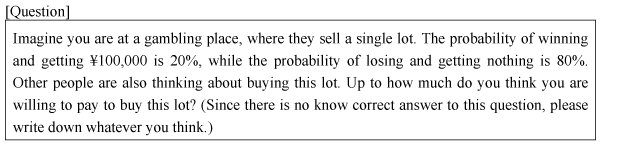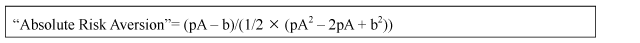# Appended Note 3-10 Calculation method for absolute risk aversion

[Toc]  [Prev]  [Next]

## 1. Outline

The absolute risk aversion was calculated based on responses to the following question asked in the "Public Opinion Poll on Consumption and Savings Behavior and The National Burden (2005)" (See Note 3-4).Specifically, the expected value of the lot is ¥20,000, obtained by multiplying the prize money by 20%. When the lot is priced ¥20,000, the same as the expected value, this position is assumed to be "risk-neutral."

Suppose that there is a person who is about to purchase this lot. If he is averse to taking the risk of losing, he will offer a price less than ¥20,000 (risk-aversion). If he is willing to take the risk, he will offer a price higher than ¥20,000 (risk-loving).

## 2. Calculation method

(1) The "absolute risk aversion" can be calculated with the following formula, where A stands for the prize money of the lot, p for the probability of winning, and b for the price of the lot offered by the respondent.(2) Based on the "absolute risk aversion" obtained from the calculating formula above, the results are classified by age group to calculate the mean.

(3) The higher value of the "absolute risk aversion" means being highly risk-aversion, while the smaller value means being risk-loving.

[Toc]  [Prev]  [Next]